CBSE Class 12 Sample Paper for 2022 Boards (MCQ Based - for Term 1)

Class 12
Solutions of Sample Papers and Past Year Papers - for Class 12 Boards

## (c) (-1/2, 5/2)  (d) (1/2 ,  5/2)

This question is inspired from Question 22 - CBSE Class 12 Sample Paper for 2021 Boards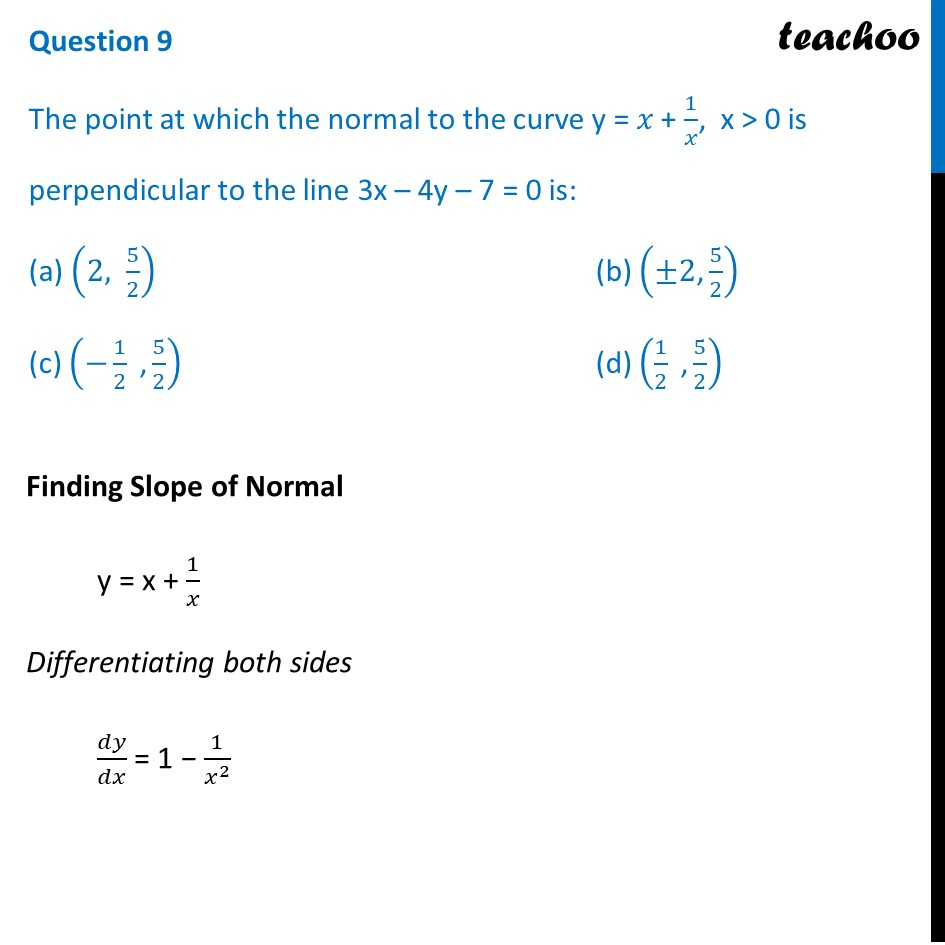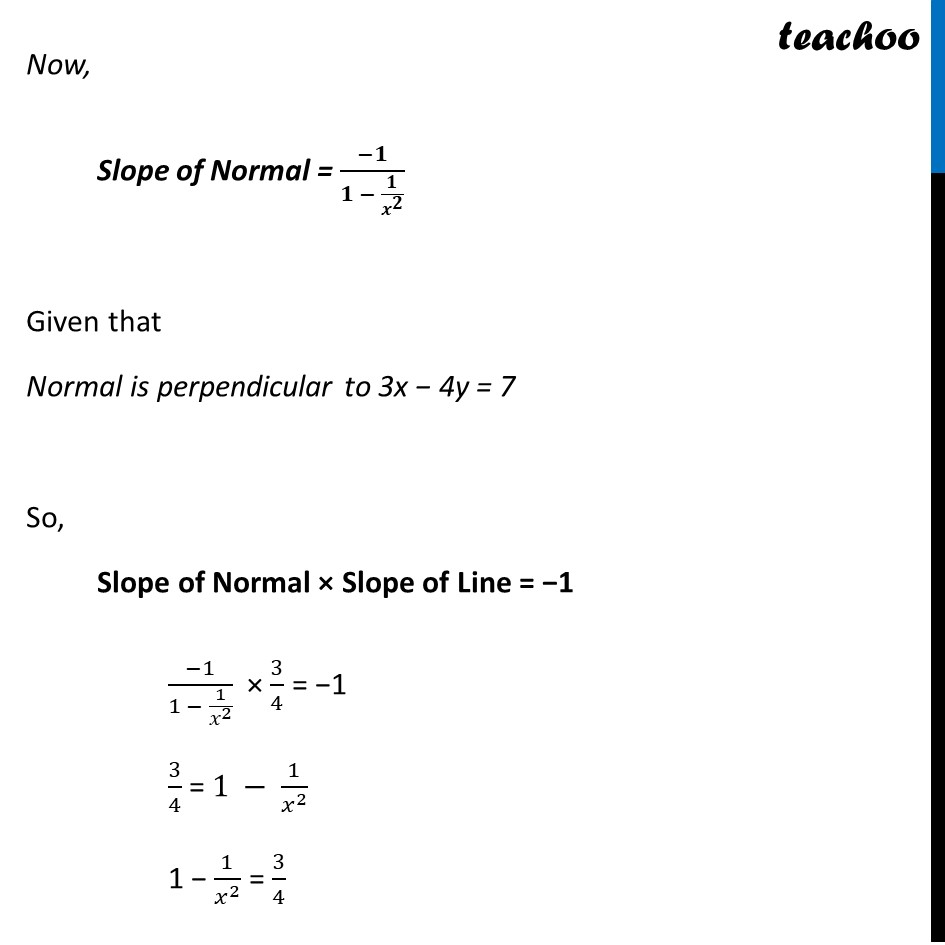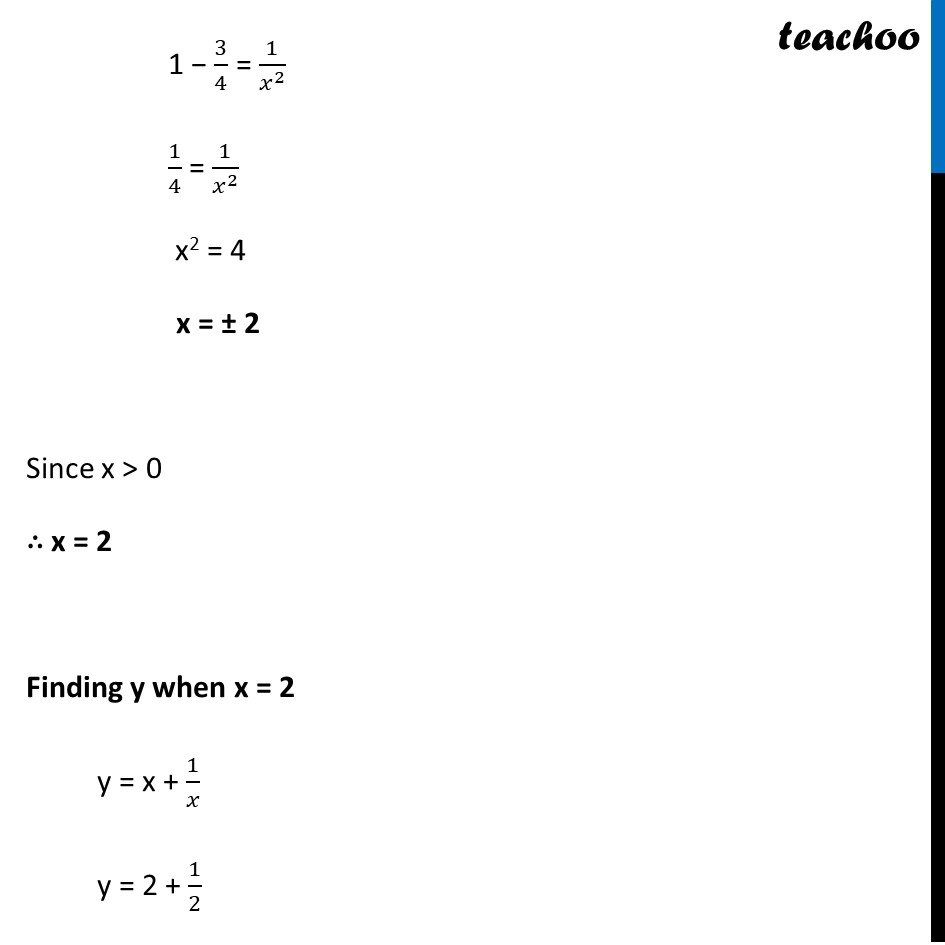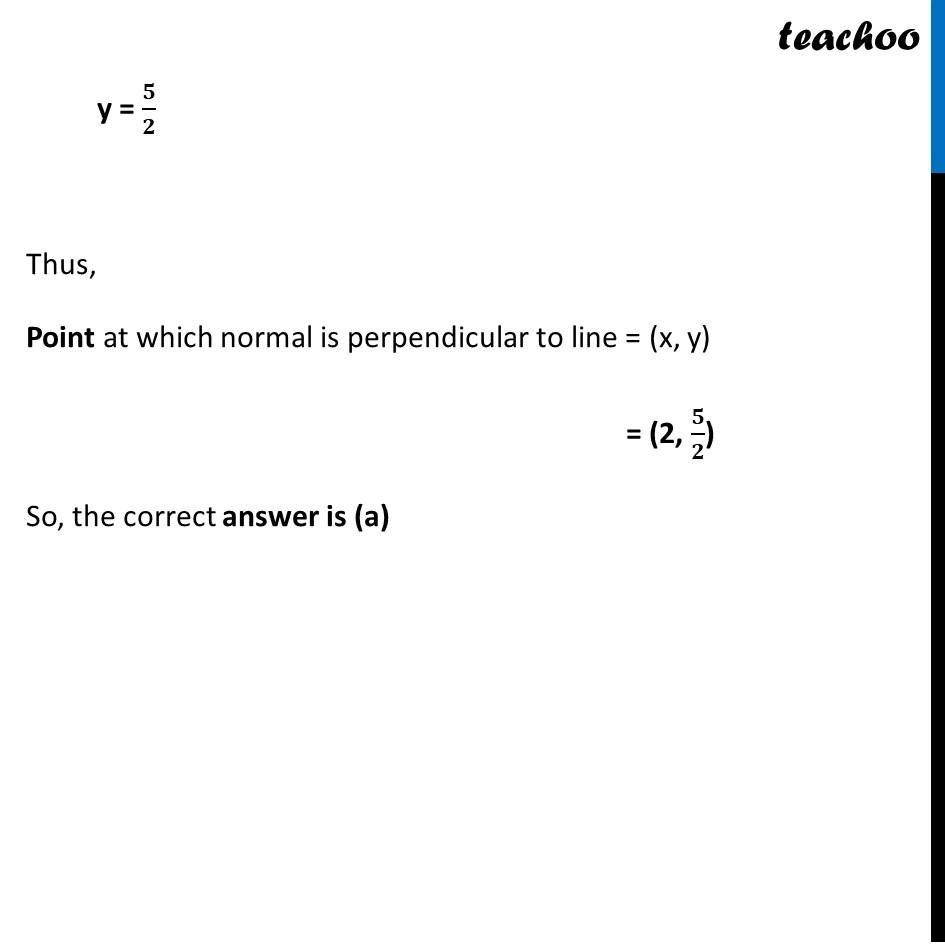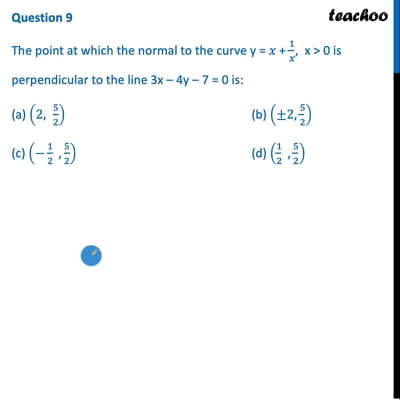This video is only available for Teachoo black users

Introducing your new favourite teacher - Teachoo Black, at only ₹83 per month

### Transcript

Question 9 The point at which the normal to the curve y = 𝑥 + 1/𝑥, x > 0 is perpendicular to the line 3x – 4y – 7 = 0 is: (a) (2, 5/2) (b) (±2"," 5/2) (c) (−1/2 " ," 5/2) (d) (1/2 " ," 5/2) Finding Slope of Normal y = x + 1/𝑥 Differentiating both sides 𝑑𝑦/𝑑𝑥 = 1 − 1/𝑥^2 Now, Slope of Normal = (−𝟏)/(𝟏 − 𝟏/𝒙^𝟐 ) Given that Normal is perpendicular to 3x − 4y = 7 So, Slope of Normal × Slope of Line = −1 (−1)/(1 − 1/𝑥^2 ) × 3/4 = −1 3/4 = 1 − 1/𝑥^2 1 − 1/𝑥^2 = 3/4 1 − 3/4 = 1/𝑥^2 1/4 = 1/𝑥^2 x2 = 4 x = ± 2 Since x > 0 ∴ x = 2 Finding y when x = 2 y = x + 1/𝑥 y = 2 + 1/2 y = 𝟓/𝟐 Thus, Point at which normal is perpendicular to line = (x, y) = (2, 𝟓/𝟐) So, the correct answer is (a)